# How Spatial Outlier Detection works

The Spatial Outlier Detection tool works by calculating a local outlier factor (LOF) to measure the degree by which points in a study area are outlying from other points in their local neighborhood. In addition to classifying input points as outliers or inliers, the tool can produce a raster surface with the calculated local outlier factor across the study area, which may assist in determining how new observations will be classified given the spatial distribution of your data. Furthermore, the tool can optimize the selection of needed parameters, such as the number of neighbors and the percent of locations considered outliers.

## Potential applications

Potential applications of this tool include the following scenarios:

• An organization maintains air quality monitoring stations that are used for air quality surface interpolation and the organization wants to identify the most isolated monitors to identify where supplemental data gathering will be necessary.
• Blood donation drives are often hosted near clusters of potential donors to minimize the travel needed by each donor, but important donors that live far away may require further communication and incentives to help spur voluntary donations. A coordinator may identify these candidate donors that are considered spatial outliers and send a mailer with additional incentives for traveling farther to a blood donation drive.

## Defining criteria for detecting spatial outliers

For the tool to measure and identify spatial outliers, it requires a value for the Number of Neighbors parameter evaluated for each feature and a value for the Percent of Locations Considered Outliers parameter in the study area; these criteria are important when determining the size of the neighborhood in the LOF calculation and the threshold for designating outliers and inliers.

• The Number of Neighbors parameter establishes a neighborhood for each feature. The LOF calculation uses this neighborhood to calculate a reachability distance and a local reachability density, which forms the basis of comparison to estimate how outlying a feature is spatially from features in its immediate vicinity.
• The Percent of Locations Considered Outliers parameter establishes a threshold for designating features as outliers or inliers. This threshold uses the calculated LOF values for all features in the input data, establishing the amount of features with the highest LOF values that are designated as outliers.

Where possible, it is recommended for domain knowledge that you help set the value of these parameters, such as in the following examples:

• A transportation engineer may have inherent domain knowledge about how many crashes in an intersection indicate a systemic safety problem and may use this value as the number of neighbors evaluated when detecting spatial outliers.
• A coordinator for a blood donation drive has a list of potential donation volunteers. The coordinator has a budget to incentivize the 10 percent most remote volunteers to compensate for their travel time to a blood donation site, and they use 10 percent for the percent of locations considered outliers to help plan the sites and incentives for the blood donation drive.

## Tool outputs

The tool provides an output feature layer highlighting the features designated as spatial outliers. Outliers are symbolized in orange, and inliers are symbolized in semitransparent gray, allowing inlier spatial density to be assessed visually.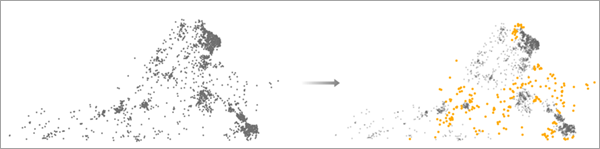The feature layer includes two charts: a bar chart showing the count of outliers and inliers and a histogram showing the distribution of LOF values.

The bar chart showing the count of outliers provides an immediate count of outliers and can be an effective way of selecting all outliers from the output analysis.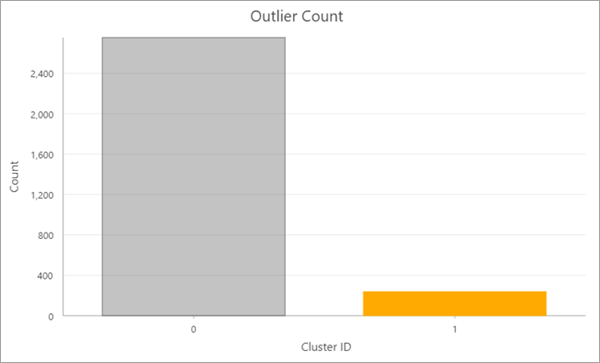The histogram showing the distribution of LOF values includes the average LOF value and the LOF threshold used to distinguish outliers and inliers.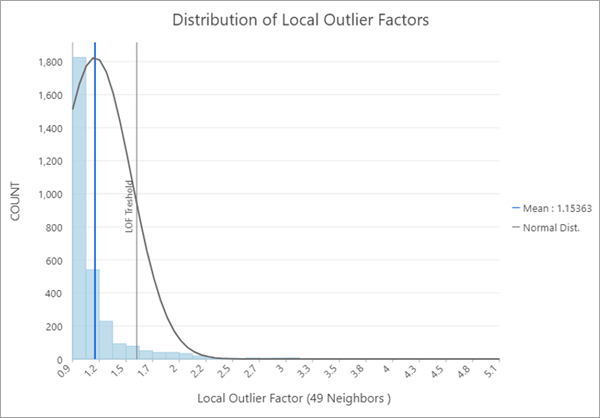Additionally, if a value is entered in the Output Prediction Raster parameter, an output raster is produced showing the calculated LOF value for each cell in the study area.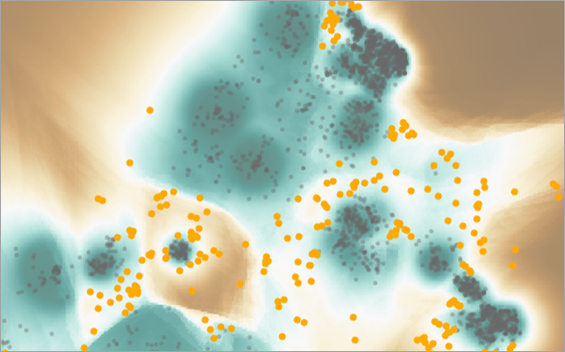## Understanding spatial outlier detection

Identifying abnormal, or outlying, locations is often more important than identifying typical, or clustered, locations. An example is the investigation of potentially fraudulent financial transactions, which often occur in abnormal locations that differ from typical spatial patterns of transactions.

Despite this need, most approaches that attempt to identify outliers focus on first identifying clusters and then using the remaining features as corollaries for spatial outliers. For example, the Density-based Clustering tool is proficient at defining and identifying spatial clustering using a variety of approaches, but its identification of outliers is relegated to features that did not satisfy the criteria for a cluster, binarily designated as noise features. Consequently, the sole use of clustering approaches to identify spatial outliers includes at least two drawbacks. First, clustering approaches, by design, are focused on defining and identifying clusters, not outliers. Second, the designation of an outlier is often done in a binary manner, without tolerance or quantified levels of how much an observation is an outlier.

The local outlier factor (LOF) addresses these drawbacks by focusing on identifying outliers and by providing a measurement of how outlying a feature may be. Furthermore, this approach uses local density patterns to compare the density of a feature's neighborhood in relation to the neighborhoods of other features in its vicinity. This allows a distinction between global outliers, points that are abnormal in the context of the entire study area, and local outliers, points that are abnormal in the context of their immediate vicinity. The focus on local outliers helps shed light on more complex local phenomena that require closer investigation, such as the transaction history scenario that was previously mentioned.

## Local outlier factor

The local outlier factor calculation is the main mechanism for identifying and describing spatial outliers. It is characterized by four main steps: establishing a neighborhood, finding the reachability distance, calculating the local reachability density, and calculating the local outlier factor itself. Each step is described in the sections below.

### Establish a neighborhood and find the reachability distance

A local neighborhood is established for each location using a specified minimum number of features. This approach is commonly referred to as K-nearest neighbors, where K corresponds to the specified minimum number of features in the vicinity of the currently analyzed feature. As an example, the illustration below displays a scenario for feature A, where the number of neighbors, k, is equal to 4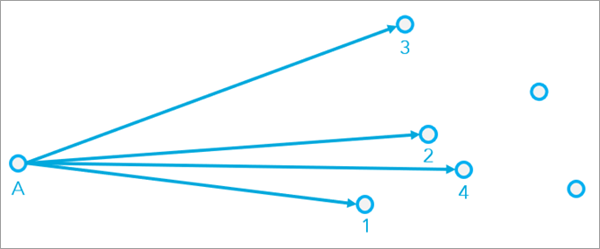Once a feature's neighborhood is established, the reachability distance corresponds to the larger of the distance between A and B and the distance from B to its kth nearest neighbor.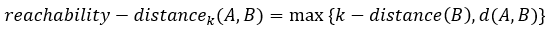The following illustration shows the reachability distance for point A in a scenario where k = 4.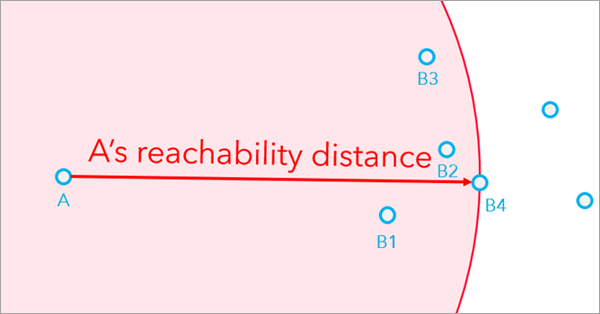In the same manner, each feature has a reachability distance defined by its K-nearest neighbors.

### Find the local reachability density

Once a reachability distance is found for each feature, the average of the reachability distances for all features in the feature's neighborhood is calculated. This average is used to determine the local reachability density, which is a measurement of spatial density for the feature's neighborhood. The calculation for the local reachability density corresponds to the inverse of the average reachability distance for all features in a feature's neighborhood.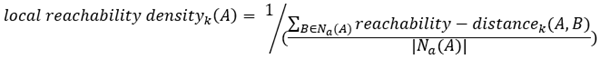Another way to conceptualize local reachability density is to calculate the reachability distance for all features, B1 through B4, that belong to the neighborhood of feature A, as shown in the image below.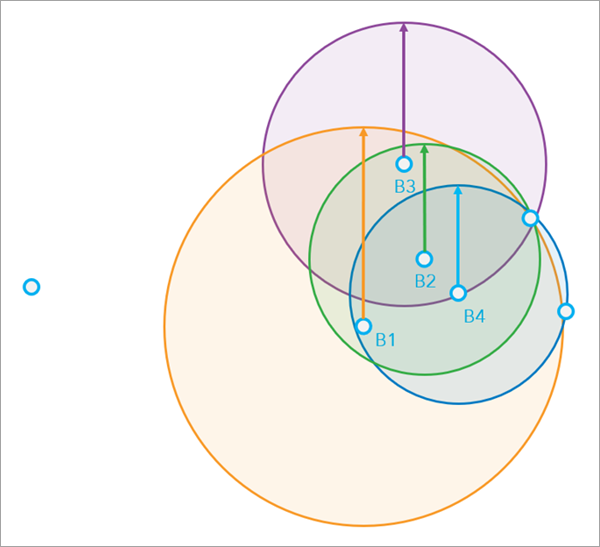Then divide the total distance by the number of features (4, in this case), and take the inverse (divide 1 by this total).

You may further conceptualize that as the average reachability distance for features increases, the local reachability density decreases. Consequently, as the average reachability distance for features decreases, the local reachability density increases.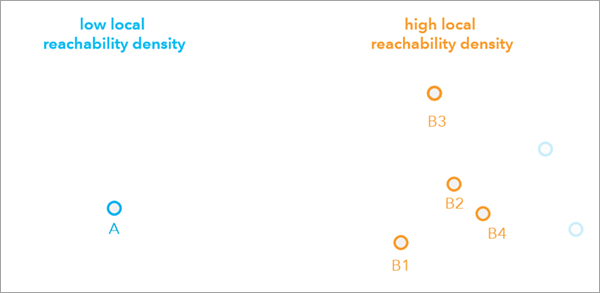### Calculate the local outlier factor

With local reachability densities calculated for all features, the final step in the local outlier factor calculation is to compute the ratios between the local reachability density of a feature and the local reachability density of each of its neighbors. The average of these ratios is the local outlier factor.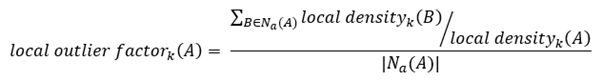To conceptualize how this helps detect whether a feature is a spatial outlier, consider that as the local reachability density of a feature decreases (in other words, the neighborhood of a feature is sparse) and the local reachability density of its neighbors increases (in other words, the neighborhood of a feature's neighbor is more dense), the local outlier factor increases: the feature is more outlying because its spatial density is low and the spatial densities of its neighboring features are higher.

With local outlier factors calculated for all features, the tool then uses the percentage of locations to be designated as outliers parameter value to designate features as outliers and inliers. Consequently, the selection of an appropriate percentage is among important criteria when defining and interpreting the analysis results.

## Considerations and interpretations of outputs

There are several important considerations when interpreting the output of this tool.

• The LOF values calculated for an input dataset cannot be used to compare with calculated LOF values in a different dataset. The LOF calculations are dependent on the spatial distribution of the input features in a dataset; consequently, any differences in separate datasets will result in different calculated local reachability densities and LOF values.
• The calculated LOF results may differ between a point in the output features and a cell in the output prediction raster coinciding with the point. The reason for this difference is that the point's neighborhood includes the neighbors in its vicinity, and does not include itself; however, the raster cell coinciding with the point includes the point as one of its neighbors.
• Small differences in values submitted for the Percent of Locations Considered Outliers parameter may result in the same output percent of locations considered outliers. This can occur when similarities in spatial distribution for features result in the same LOF value for multiple features and the same LOF threshold is established even if the percent is different by a small margin.
• Consider a simple dataset with 10 features whose LOF calculation results in the following LOF values: [0, 1, 2, 3, 4, 5, 9, 9, 9, 9]. In this example, a value of 10 percent for percent of locations considered outliers would result in selecting the top 10 percent LOF values, which corresponds to an LOF threshold of 9. Similarly, passing a value of 40 percent for percent of locations considered outliers would result in selecting the top 40 percent LOF values, though this will still set an LOF threshold of 9. Therefore, the output count of outliers designated as outliers will be the same for percentages 10 percent through 40 percent.